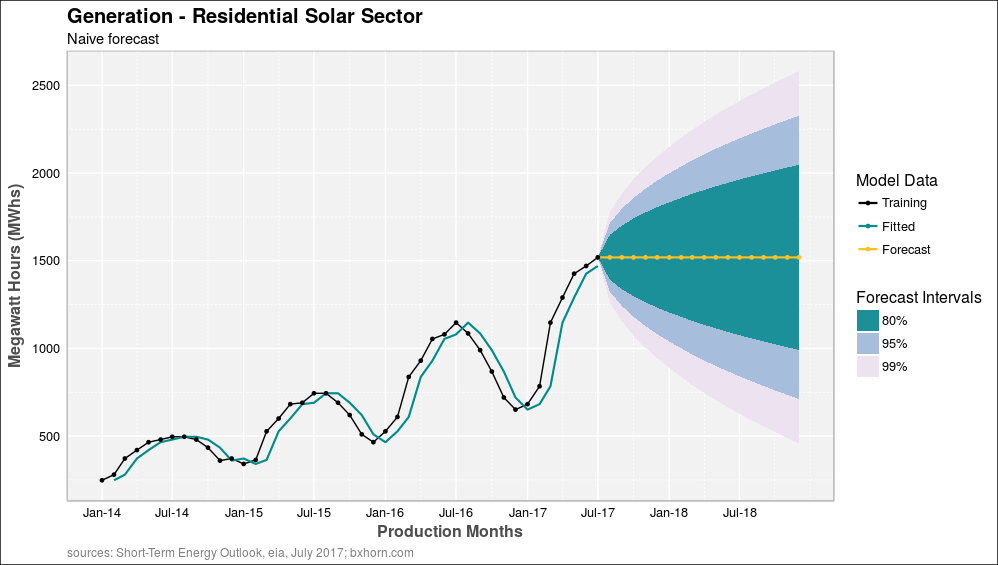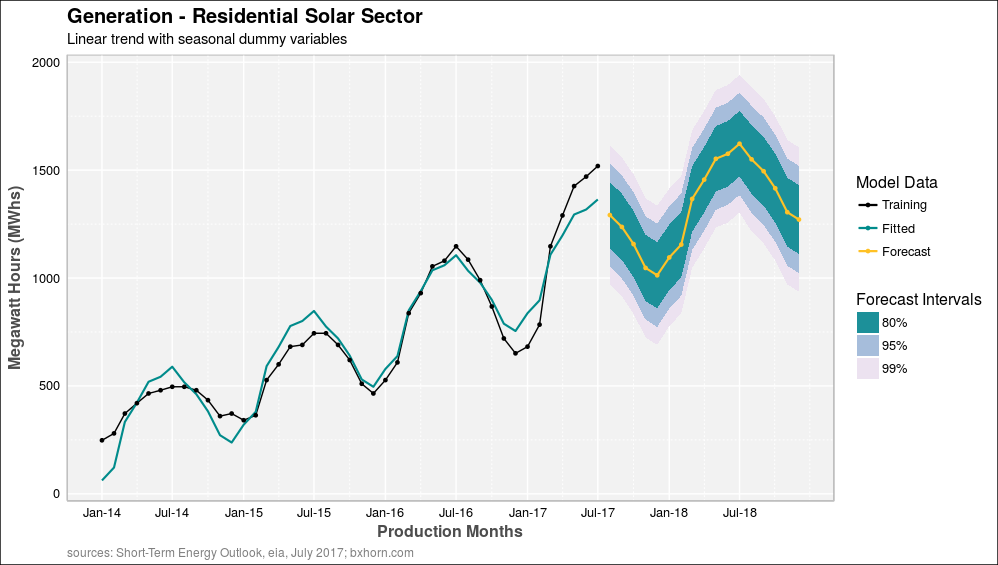# Plotting Forecast Data Objects Using ggplotRobert Hyndman is the author of the forecast package in R. I’ve been using the package for long-term time series forecasts. The package comes with some built in methods for plotting forecast data objects in R that Ive wanted to customize for improved clarity and presentation.  The following article achieves that goal and shares two scripts for plotting forecast data objects using ggplot.

#### Script structure

The first script is a custom plotting theme to configure the R graphical device for custom data visualization. The template changes the default plot theme for ggplot and replaces it with the Goggle Docs graph format and some custom label options.  The result is a clean plot format and new default template for repeated use.

The second script is a custom function for plotting forecast data objects that utilizes the custom theme.  The function presents training, fitted and forecast data along with 3 predictive forecast intervals (80%, 95% and 99%).  The function should work with any of the forecast model types for time series data including:

• basic or naive forecast methods
• models that use seasonal dummy variables
• trigonomic models using Fourier series
• exponential smoothing models
• autoregressive moving average models (ARIMA) and
• neural network models

Each of these model types can be easily accommodated since the forecast package utilizes one format for forecast data output and easily supports the required output for 3 predictive intervals.

#### Custom function for plotting forecast() objects in ggplot

By way of example, the function can be tested using the following time series data for small-scale residential solar production for the US:

The data is first assigned a variable name (x) and converted into a time series data object (res.gen) using the ts() function with monthly frequency.  A naive forecast model (naive.fx) is generated using a a random walk forecast with a forecast horizon (h) of 17 months and 3 predictive intervals (80%, 95% and 99%).  The resulting plot of the forecast data object using is pictured at the start of this article.  The naive forecast is easily recognized as one where future values equal the most recent observed value:

Using the same data, a more realistic forecast can be obtained by capturing the historic data trend and seasonal pattern.  This is accomplished by defining a linear model (fit.y) with seasonal dummy variables.  The model results are then converted to a forecast data object (fx.y) with the same forecast horizon (h = 17) and predictive intervals.  The results are pictured below:In this case, the fitted values capture the trend and seasonal swings in the power generation training data, but the fitted value at the end of the training period is below the actual data.  As a result, the forecast data begins below the actual data.  Nevertheless, the observed trend and seasonal pattern are still projected forward by the linear model with seasonal dummy variables.  This is sufficient for testing the plotting function.  Later articles with explore more accurate forecast methods.

This entry was posted in Data Science, ggplot2, Modeling, R Programming. Bookmark the permalink.